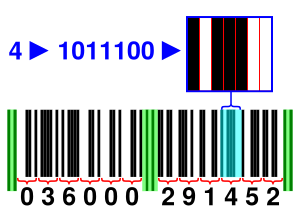# Checksum facts for kids

Kids Encyclopedia FactsA modern EAN barcode: The parts in green are called spacers: They separate the groups of numbers. Each number is coded by 7 bits, as shown. The first 11 digits carry the product number. The final digit, in this case the number "2", is the checksum. The checksum is added so that the sum of the even positions, and that of the odd ones multiplied by three, modulo 10 is 0.

A checksum is a number. It is a kind of redundancy check. There are different ways to calculate it. It serves as a check, that no errors have been made when writing down the number. In its simplest form, the digits are simply added up. This can however not detect errors of swapping digits around. Better ways (algorithms) of calculating a checksum are to use a Hamming code, a cyclic redundancy check or modular arithmetic. One of the uses of checksums is to check that account numbers have been entered correctly.

Portuguese bank account identifiers are a good example of how checksums work. They have 21 digits. The first 19 digits identify the bank, branch and account number. The last two digits are "mod 97" checksum digits based on modular arithmetic. A typical Portuguese bank account number is 0002.0123.12345678901.54. The digits have the following meanings:

• The digits "0002" represent the bank.
• The digits "0123" represent the branch of the bank.
• The digits "12345678901" represent the account number
• The digits "54" represent the checksum.

If 000201231234567890154 (the bank account number without the dots) is divided by 97, the answer is 207458809978249 remainder 1. Since the remainder is "1", the bank account identifier is probably correct. If the person writing down the number missed a digit, swapped two digits around or wrote a digit down incorrectly the remainder would not have been "1". If this is the case then the computer should detect an error and give an error message.

Another place where checksums are used are serial numbers for computer software.Checksum Facts for Kids. Kiddle Encyclopedia.